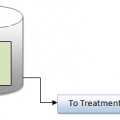## Basic Pumping Calculations

BASIC PUMPING CALCULATIONS
Certain computations used for determining various pumping parameters are important to the water/wastewater operator. In this section, we use basic-pumping calculations relevant to the subject matter.
PUMPING RATES
Important Point:
\$The rate of flow produced by a pump is expressed as the volume of water pumped
during a given period.The mathematical problems most often encountered by water/wastewater operators when determining pumping rates are often determined by using Equations

Pumping rate (gpm)=gallons / minutes

Pumping rate (gph)=gallons / hours

Example:
Problem
The meter on the discharge side of the pump reads in hundreds of gallons. If the meter shows a reading of 110 at 2:00 p.m. and 320 at 2:30 p.m., what is the pumping rate expressed in gallons per minute?
Solution
The problem asks for pumping rate in gallons per minute (gpm), so we use Equation
• Step 1: To solve this problem, we must first find the total gallons pumped (determined
from the meter readings).
32,000 gal – 11,000 gal = 21,000 gal
• Step 2: This quantity was pumped between 2:00 p.m. and 2:30 p.m. for a total of 30
minutes. From this information, calculate the gpm pumping rate:

Pumping rate (gpm)=21,000 gal / 30 min
=700 gpm pumping rate## Author:T.P.Sivanandan

#### Why Fine Bubbles are Better than Coarse bubble in Aeration Tank#### How to Calculate Lime Dosage Requirement in Sedimentation Process## 1 thought on “Basic Pumping Calculations”

1.Prakash Deshmukh says:

Dear Sir,
very good content. Would like to give suggestion that in pumping calculation M3/Hour or LPM for small capacity pumps will be more appropriate than GPM as now the unit is lpm or m3/hour.
Thanks & Regards,